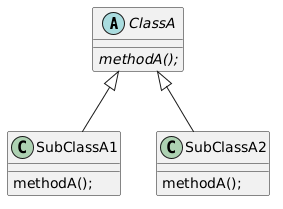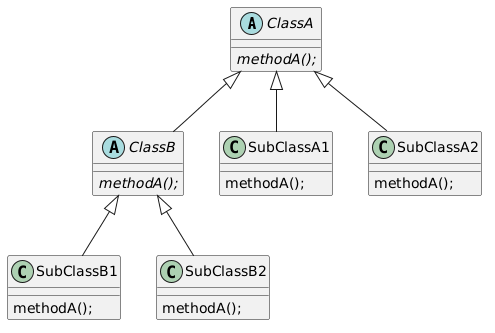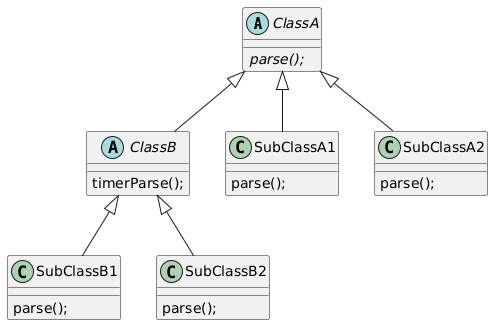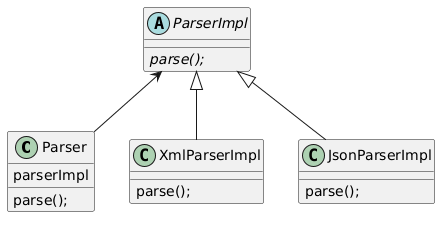Now that I need to write some Java code at my work place, I’ve started re-learning Java’s typical design patterns. I’m going to summarize what the “Bridge Pattern” is in this article.

Let’s imagine that a class is inherited by 2 classes to implement an abstract method in the super class. More concretely, we can draw a diagram for that as follows:Let’s assume that we want to implement a new feature for `methodA()` and revise the method by overriding it. We can’t just add a new class to override the method. It’d end up like following:We already have a concrete method `methodA()` in `SubClassA1` and `SubClassA2` for `ClassA`, so we need the counterparts of them for `ClassB` as well (They’re expressed as `SubClassB1` and `SubClassB2` in the diagram above).

The whole architecture gets complicated unnecessarily. That’s why we want to introduce “Bridge Pattern” to make it simple.

## Concrete Example

Let’s assume that we’re trying to create a `Parser` class. We want to parse `XML` and `JSON`.

``````public abstract class ClassA {
public abstract DataObj parse(String txt);
}
``````
``````public class SubClassA1 extends ClassA {
public DataObj parse(String xmlTxt) { /* Let's assume that this one parses XML string */ }
}
``````
``````public class SubClassA2 extends ClassA {
public DataObj parse(String jsonTxt) { /* Let's assume that this one parses JSON string */ }
``````

Now, what would you do if you want to add a feature to measure the time to run `parse()` in `ClassA`. The easiest way is subclassing `ClassA` and override `parse()` as follows:

``````public abstract class ClassB extends ClassA {
public DataObj timerParse(String txt) {
long start = System.currentTimeMillis();
DataObj dataObj = parse(txt);
long end = System.currentTimeMillis();

System.out.println("time:"+(end - start));
return dataObj;
}
}
``````

Here’s a big problem: To use `timerParse()`, `parse()` method needs to be re-implemented in the subclasses of `ClassB`, because `SubClassA1 ` and `SubClassA2` are the subclasses of `ClassA`, not `ClassB`.

In other words, we’d need to implement following stuff:This is ridiculous. How come we need to implement 4 concrete `parse()` methods when we just want to have 2 types of parsers (one is for XML, and the other one is for JSON).

## Bridge Pattern is here to help

Here’s how to adopt Bridge Pattern:

1. Create a class that has an “implementation object” as a member (see below)
2. Create an abstract “Impl (Implementation)” class that inherits the class
3. Create concrete classes that inherits the “Impl” class and override methods in it

For our case, the architecture would look like following:With this architecture, `Parser` class can be implemented as follows:

``````public class Parser {
private ParserImpl parserImpl;
public Parser(ParserImpl parserImpl){
// parserImpl can be an object of XmlParserImpl or JsonParserImpl
this.parserImpl = parserImpl;
}

public void parse(String txt){
parserImpl.parse(txt);
}
}
``````

Apparently, `ParserImpl` is working as a proxy (a.k.a. Bridge) so `Parser` can transparently accept objects of any subclasses of `ParserImpl`.